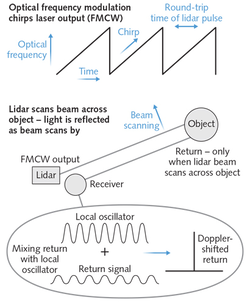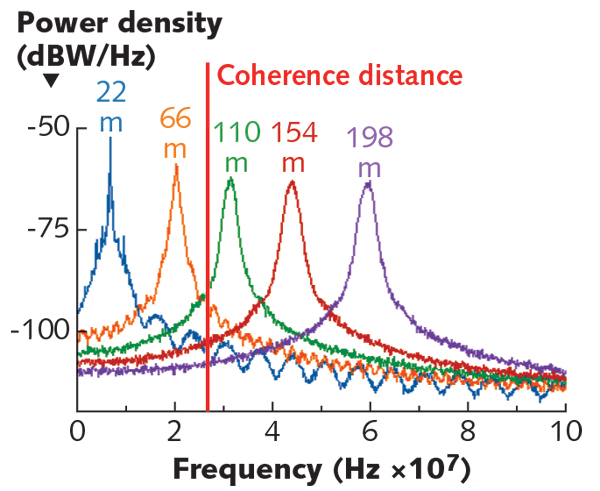# Time of Flight vs. FMCW LiDAR

AEye.ai shared comparison of time of flight (ToF) and Frequency Modulated Continuous Wave (FMCW). I had worked in ToF when I was in Samsung (2014~2016) and am very interested in learning more of it.

# ToF Lidar

The principal of ToF lidar can be described by this figure from WikipediaToF works by sending a pulse of light and measure the delay $$\Delta t$$ to the receiving of this pulse.

$d=\frac{c\Delta T}{2}=\frac{ct}{2}\frac{q_1}{q_1+q_2}$

Here c is the speed of light, $$q_1$$ and $$q_2$$ is the reading of light energy. The pulse width (t) affects the working range and resolution of the system. The smaller t, the higher depth resolution and smaller working range. There are limitation of this system:

• the pulse is perfect square wave, which is unlikely feasible in high frequency system
• the impact of ambient is very low compare with the light source itself. This is usually acheived with a high engery short pulse (5~10ns) laser.

To address those issues, continuous-wave method is used. Here instead of using square wave, sinusoid wave is used, we capture reading of received light source as in four phases. Then the distance is computed as:

$d=\frac{ct}{2}\arctan{\frac{q_3-q_4}{q_2-q_1}}$

Here $$q_3-q_4$$ and $$q_2-q_1$$ canceled out the impacts of ambient light.

# FMCW Lidar

The princpial of FMCW lidar can be described as figure below (from Laser World). The light source is frequence modulated chrips (i.e., signal with changing frequency). To compute the distance, we compute the cross-correlation of the signal source to the received signal and the location of peak is linear to the distance to the object.$co(x,y,\Delta)=\int_t{x(t)\times y(t+\Delta)\delta_t}$

Here x is the source signal and y is the received signal. It is known that the cross-correlation of two signals will be zero if the frequency of two signals doesn’t match. This figure illustrates the correlation for objects at different distances.# ToF vs FMCW

Item ToF FMCW
Working Distance [now] eye-safety power restrictions that limit lidar range to 60–100 m. Shifting to the retinal-safe 1550 nm band allows pulse powers high enough to range from 200 to 300 m Existing FMCW lidars are limited to coherence lengths around 100 m, which could restrict their range to about 50 m
Maturity Higher Lower
Complexity Low. High. Need to modulate the light source (a tunable laser with good polarization control and very long coherence length) and complex signal processing
Cost Low. Could be even lower with solid state scanning Higher (higher cost in light source and signal processing but cheaper in sensor).
Interference Low surface reflection, secondary reflections, other Lidar system less sensitive to low surface reflection, secondary reflections, other Lidar system
Velocity Indirectly computed from distance Direct measurement by doppler shift in frequency.

# Reference

• https://www.aeye.ai/whitepapers/time-of-flight-vs-fmcw-lidar-a-side-by-side-comparison/
• https://3da.medium.com/lidar-fmcw-vs-tof-lidar-8288663bd73
Written on February 21, 2021